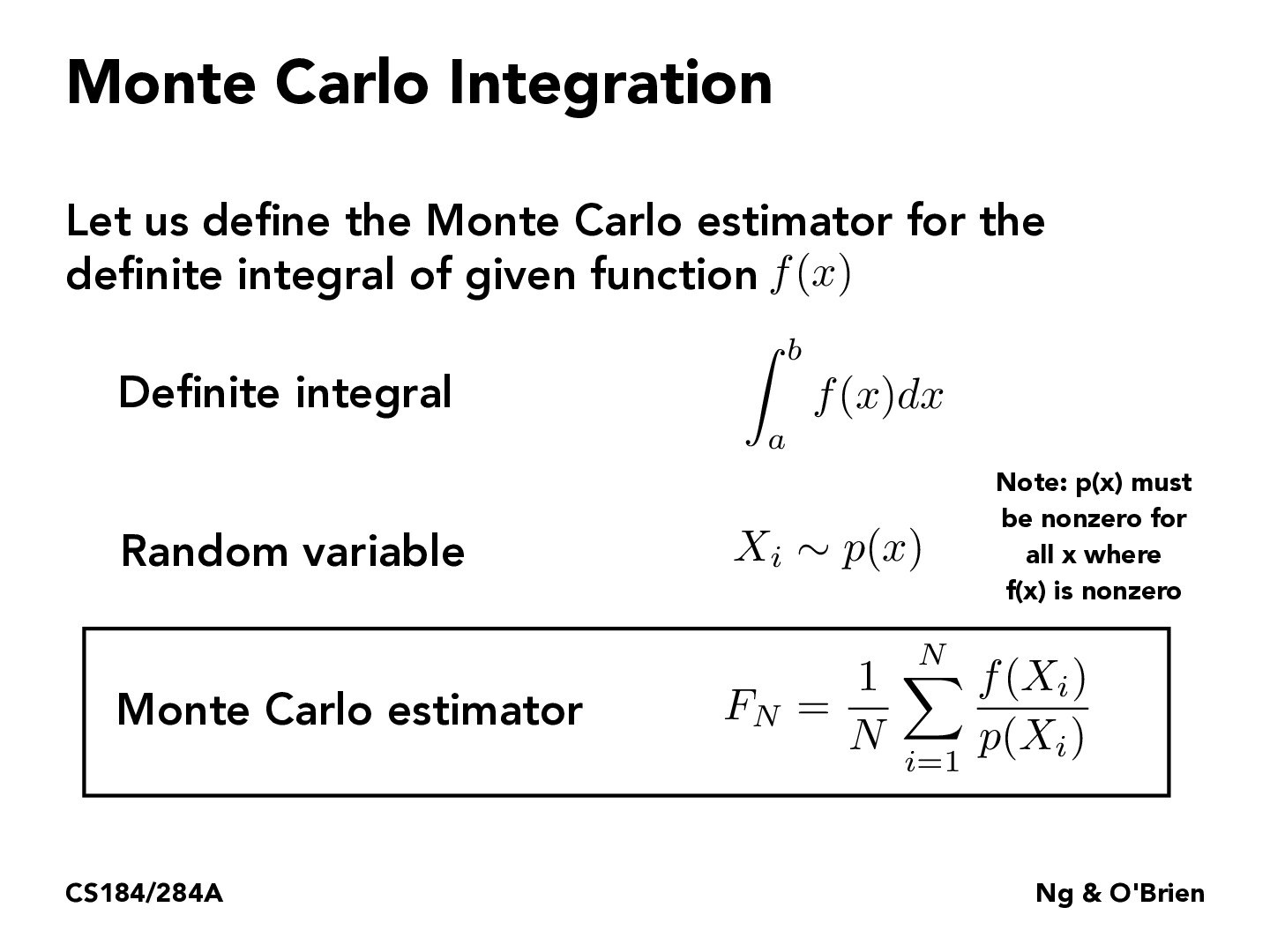Lecture 12: Monte Carlo Integration (21)starptr

What's the intuition behind why we divide by the probability in the sum? My intuition makes me think that we should be multiplying instead since if a sample $f(X_1)$ is very likely to appear, it should be weighted more since we're estimating the true valueZc0in

@starptr Firstly, p is less than 1, so if you multipy it, the F_N will definitely nuch less than the origin. Secondly, if X_i has large p, which means it will more likely to appear in our sampling more than once, so for the single f(x_i). it should be weighted less.jonathanlu31

My interpretation is that since p(x) is just any arbitrary random variable, if p(x) samples xi a lot, that doesn't mean f(xi) is closer to the true value. If the estimator didn't "normalize" by dividing by the probability of sampling xi, then xi's that are more likely to be sampled by this arbitrary variable would have more influence on the estimate than they should. Samples that are selected less should be weighted more since the estimator doesn't get to see them as much, and vice versa.bbcd0921

How can Monte Carlo integration be used to estimate the value of a multi-dimensional integral, and what factors can affect the accuracy of the estimation?

You must be enrolled in the course to comment